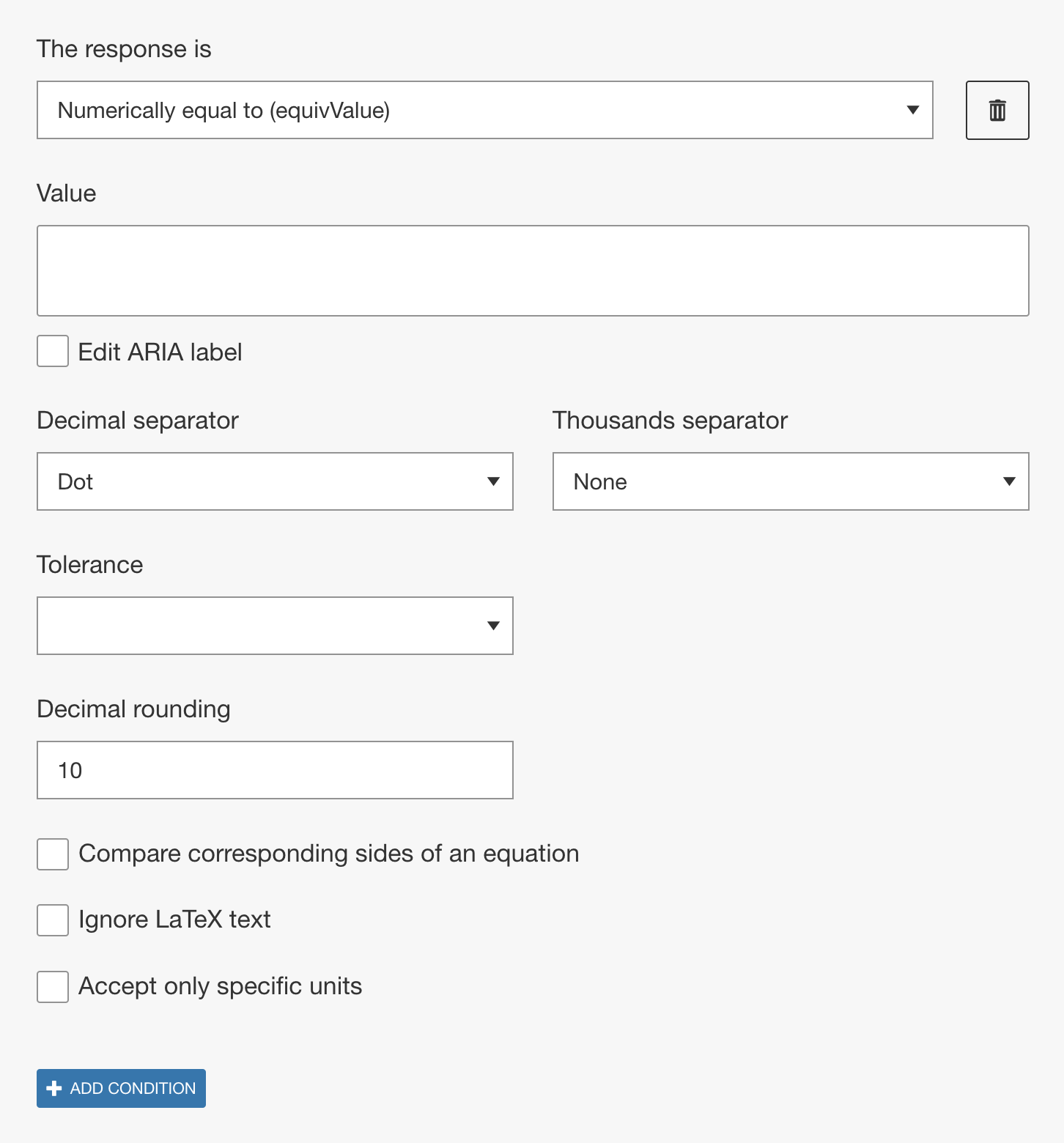# Numerically equal to (equivValue)

## Overview

The Numerically equal to (equivValue) method checks whether the learner response is numerically equal to the sample correct answer set by the author. This method allows for values to be represented in different ways including using different units of measurement, when applicable.

Although similar to equivSymbolic since both methods do not require a specific form, the equivValue method cannot be used with variables. It does, however, work with numeric values, like decimals, fractions, and percentages, and numeric values including units of measurement. There are also additional options available for setting a tolerance.• Decimal and thousands separator
Authors can specify what separators should be recognized by the engine and can be used by the learner. From the Decimal separator drop-down either a dot or a comma can be selected. Depending on the selection of the decimal separator, from the Thousand Separator drop-down dot, comma, and/or space can be selected.
• Tolerance: Range between
This option allows the learner response to be within or equal to a specified minimum and maximum value. The values for the lower and upper limits can be an integer or a decimal.
• Tolerance: Absolute error
This option allows the learner response to be within an error margin of plus/minus n of the authored value. Therefore the learner response will be marked correct if the absolute value of the difference of the authored value and learner response is less than or equal to n. The numerical discrepancy, n, can be an integer or a decimal.
• Tolerance: Percent error
This option allows the learner response to be within n% percent of the authored value. The percentage error, n, can be an integer or a decimal.
• Decimal rounding
This option specifies the number of decimal places the learner response and the Author value are rounded to before comparison. The maximum and default value is 10.
• Compare corresponding sides of an equation
With this option enabled, when comparing two equations the engine will compare the corresponding sides of each equation individually rather than the whole equations. For example, if the authored value was `y=x+1` and the option was selected, `y-x=1` would no longer be a correct learner response.
• Ignore LaTex text
This option refers to LaTeX text only and when enabled the engine will ignore any LaTeX text in the authored value and learner response when comparing for equivalency. For example, any text added in a Text block by the author or units entered by the keypad are set as LaTex text and would be ignored.
• Accept only specific units (Coming soon, on developer version)
Without this option enabled, the default behavior is when the authored value contains a unit of measurement the engine will accept an equivalent answer from the learner expressed with a different unit of measurement. When this option is enabled, you can require specific units by inputting the units of measurement that should be accepted in correct answers in the Accepted units field. You can enter multiple units of measurement as a comma separated list. For example if the authored value is `1 m` and accepted units was set as `m, cm`, then `1 m` and `100 cm` would be accepted correct answers but `1000 mm` would be marked incorrect.